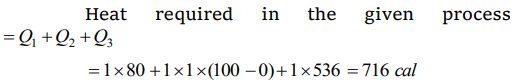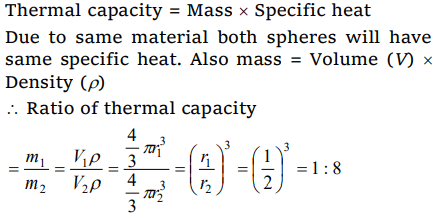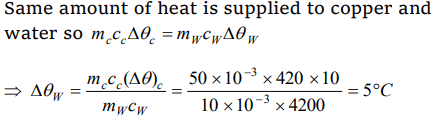## Thermal Properties of Matter Questions and Answers Part-8

1. How much heat energy is gained when 5 kg of water at 20°C is brought to its boiling point
a) 1680 kJ
b) 1700 kJ
c) 1720 kJ
d) 1740 kJ

Explanation:2. Melting point of ice
a) Increases with increasing pressure
b) Decreases with increasing pressure
c) Is independent of pressure
d) Is proportional to pressure

Explanation: Melting point of ice decreases with increase in pressure (as ice expands on solidification).

3. Heat required to convert one gram of ice at 0°C into steam at 100°C is $\left(L_{steam}=536 cal/gm\right)$
a) 100 calorie
b) 0.01 kilocalorie
c) 716 calorie
d) 1 kilocalorie

Explanation: Conversion of ice (0°C) into steam (100°C) is as follows4. 80 gm of water at 30°C are poured on a large block of ice at 0°C. The mass of ice that melts is
a) 30 gm
b) 80 gm
c) 1600 gm
d) 150 gm

Explanation: If m gm ice melts then
Heat lost = Heat gain
80 * 1 * (30 - 0) = m * 80
m = 30 gm

5. The saturation vapour pressure of water at 100°C is
a) 739 mm of mercury
b) 750 mm of mercury
c) 760 mm of mercury
d) 712 mm of mercury

Explanation: At boiling point saturation vapour pressure becomes equal to atmospheric pressure. Therefore, at 100°C for water. S.V.P. = 760 mm of Hg (atm pressure)

6. Two spheres made of same substance have diameters in the ratio 1 : 2. Their thermal capacities are in the ratio of
a) 1 : 2
b) 1 : 8
c) 1 : 4
d) 2 : 1

Explanation:7. Work done in converting one gram of ice at –10°C into steam at 100°C is
a) 3045 J
b) 6056 J
c) 721 J
d) 616 J

Explanation: Ice (–10°C) converts into steam as follows
(ci = Specific heat of ice, cw = Specific heat of water)8.If mass energy equivalence is taken into account, when water is cooled to form ice, the mass of water should
a) Increase
b) Remain unchanged
c) Decrease
d) First increase then decrease

Explanation: When water is cooled at 0°C to form ice then 80 calorie/gm (latent heat) energy is released. Because potential energy of the molecules decreases. Mass will remain constant in the process of freezing of water.

9. Compared to a burn due to water at 100°C, a burn due to steam at 100°C is
a) More dangerous
b) Less dangerous
c) Equally dangerous
d) None of these

10. 50 gm of copper is heated to increase its temperature by 10°C. If the same quantity of heat is given to 10 gm of water, the rise in its temperature is ( Specific heat of copper = 420 $Joule-kg^{-1}°C^{-1}$   )